Next: Conclusion Up: Synthetic and field data Previous: Synthetic and field data

## Land data example

Figuredisplays a CMP gather from a land acquisition. This gather is contaminated by a strong ground-roll with weak signal. The goal is to test the two proposed techniques with the same operators as in the preceding example, i.e., hyperbolic radon transform to obtain velocity information and a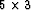PEF for the filtering and modeling of the noise.amoco
Figure 7
A CMP gather from a land survey. The ground-roll is very strong throughout the section.By doing inversion without taking care of the coherent noise, the velocity panel in Figurea is obtained. The model space is contaminated with strong noise coming from the residual in Figurec. When noise is present in the data, least-squares with a simple damping can help to mitigate the noise effects. This was not done in this case, however. The reconstructed data in Figureb is relatively noise free, which indicates that most of the energy in the model space (Figurea) is in the null space of the operator.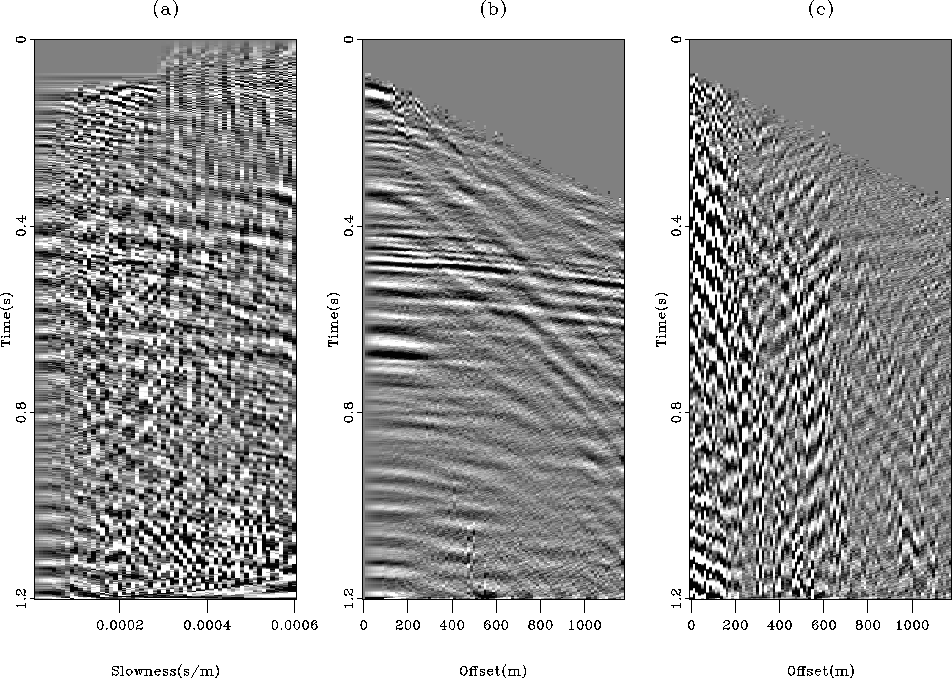amoco-nopef
Figure 8
Result of least-squares inversion of the data in Figure. (a) Estimated model space. The noise level is too high to see any useful information. (b) The remodeled data. The low noise level in this panel indicates that most of the energy in (a) resides in the null space of the operator. (c) Data residual. The residual is not IID.For the filtering and modeling techniques to work, a noise model is needed. As suggested in a preceding section, the noise model is estimated from the residual of least-squares inversion assuming that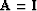(i.e., Figurec). From this noise model, aPEF is estimated. Figurea displays the result of the inversion after 40 iterations of CG. The few hyperbolas present in the data are clearly mapped in the model space without any artifact. The remodeled data in Figureb show almost no ground-roll remaining. The unweighted residual in Figurec () contains only coherent noise and the residual in Figured is almost IID: a little bit of ground-roll is still present.c-amoco
Figure 9
Inversion result with the filtering technique. (a) Estimated model. (b) Remodeled data. (c) Unweighted residual, showing the ground-roll only. (d) Residual. The residual is not exactly IID. Some coherent energy remains.The modeling technique leads to similar results to the filtering technique, as shown in Figure. The main difference comes from the residual in Figured. With the modeling method, the residual is smaller than with the filtering technique and is IID. As a final comparison between the two techniques, Figureshows how the objective function (normalized) decreases in both cases. The modeling technique has the best convergence properties.compsa
Figure 10
Inversion result with the modeling technique. (a) Estimated model. (b) Remodeled data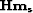.(c) Remodeled noise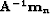.(d) Residual. The residual is IID.amococonv Figure 11 A comparison of convergence between the filtering and modeling approach for the land data example.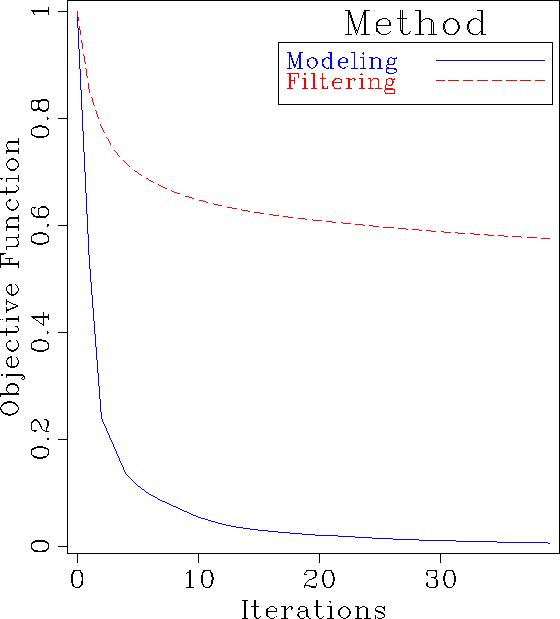Next: Conclusion Up: Synthetic and field data Previous: Synthetic and field data
Stanford Exploration Project
5/5/2005